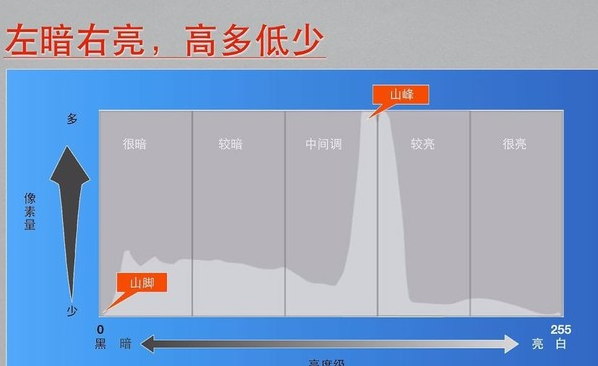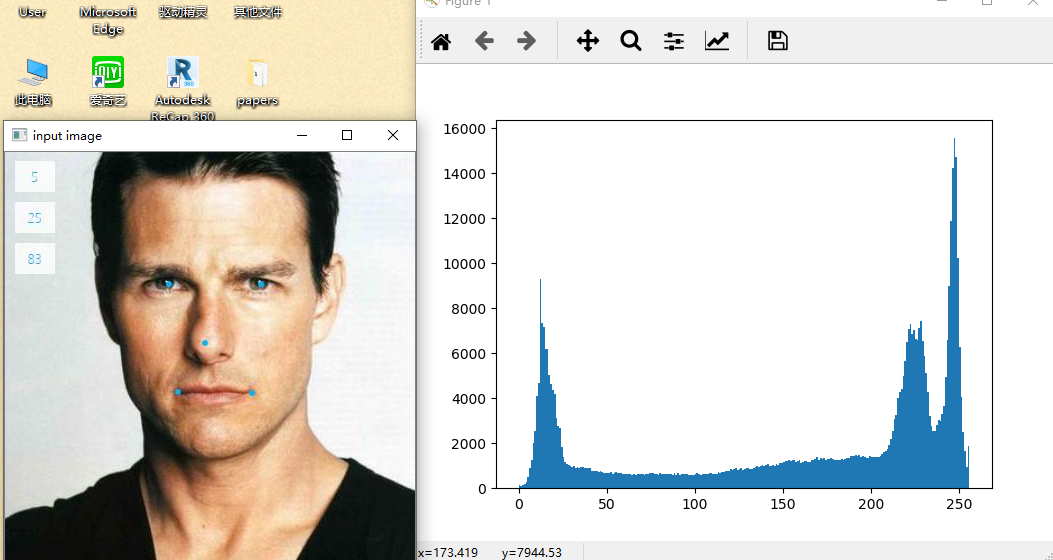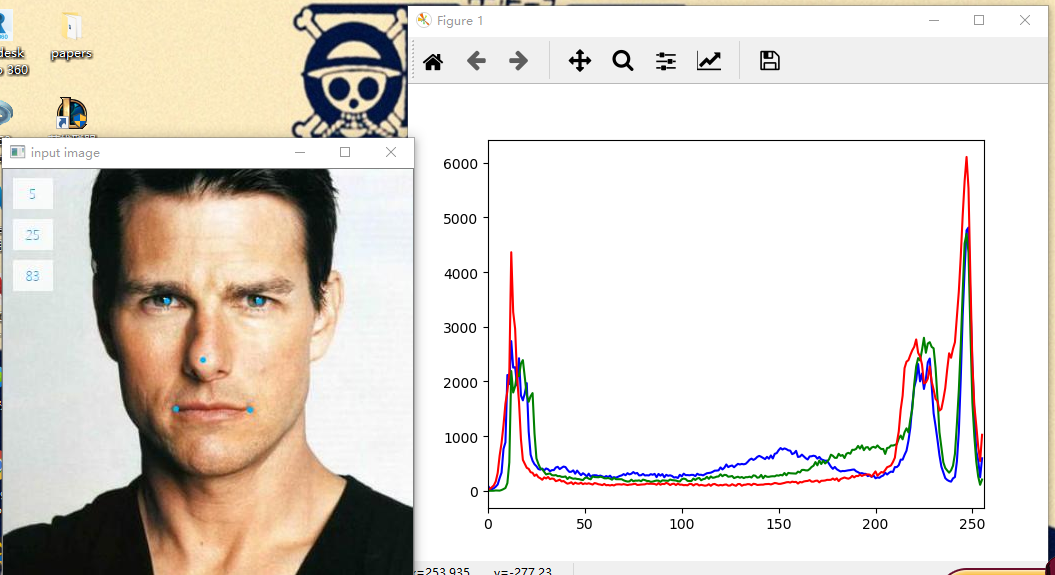# 图像处理直方图的bin

## 《数字图像处理》--直方图处理

2016-10-08 17:33:23 taoyanqi8932 阅读数 15039
• ###### 图像直方图统计

掌握OpenCV核心模块，熟练使用相关API 理解各个API背后的相关算法原理，每个参数意义 有能力解决使用应用场景问题，大量工程代码经验分享 掌握图像处理与视频分析，图像分析与测量编码与开发技巧

159人学习 贾志刚
免费试看

## 直方图### 直方图的性质：

1. 直方图反映了图像中的灰度分布规律。它描述每个灰度级具有的像元个数，但不包含这些像元在图像中的位置信息。
2. 任何一幅特定的图像都有唯一的直方图与之对应，但不同的图像可以有相同的直方图。
3. 如果一幅图像有两个不相连的区域组成，并且每个区域的直方图已知，则整幅图像的直方图是该两个区域的直方图之和

### 直方图的应用：

1. 对于每幅图像都可做出其灰度直方图。
2. 根据直方图的形态可以大致推断图像质量的好坏。由于图像包含有大量的像元，其像元灰度值的分布应符合概率统计分布规律。假定像元的灰度值是随机分布的，那么其直方图应该是正态分布。
3. 图像的灰度值是离散变量，因此直方图表示的是离散的概率分布。若以各灰度级的像元数占总像元数的比例值为纵坐标轴做出图像的直方图，将直方图中各条形的最高点连成一条外轮廓线，纵坐标的比例值即为某灰度级出现的概率密度，轮廓线可近似看成图像相应的连续函数的概率分布曲线## 直方图均衡化1. 统计原图像每一灰度级的像元数和累积像元数。
2. 按下图公式计算变换后的值
3. 四舍五入得到新的灰度值
4. 统计像元``````clc;clear;close all;
subplot(1,2,1);
imshow(I)
title('原始图像')
subplot(1,2,2)
imhist(I);
title('直方图')
J=histeq(I);
figure;
subplot(1,2,1)
imshow(J)
title('直方图均衡化')
subplot(1,2,2)
imhist(J)
title('直方图')````````````#include "opencv2/opencv.hpp"
#include "opencv2/opencv_modules.hpp"
#include "opencv2/highgui/highgui.hpp"
#include<iostream>
using namespace cv;
using namespace std;

/*
计算直方图的函数
返回的是直方图的矩阵形式
输入是图像
*/
Mat histogramCal(const Mat& image) {
int histSize = 255;                //直方图的最大像素值
float range[] = { 0,256 };
const float* histRange = { range };
vector<Mat> bgr;                   //存储图像的矩阵
split(image, bgr);                 //将彩色图像分割成，b,g,r分别存储
bool uniform = true, accumulate = false;
Mat b_hist, g_hist, r_hist;
//分别计算各个波段的直方图
calcHist(&bgr, 1, 0, Mat(), b_hist, 1, &histSize, &histRange, uniform, accumulate);
calcHist(&bgr, 1, 0, Mat(), g_hist, 1, &histSize, &histRange, uniform, accumulate);
calcHist(&bgr, 1, 0, Mat(), r_hist, 1, &histSize, &histRange, uniform, accumulate);

//绘制直方图
int hist_w = 512, hist_h = 400;
int bin_w = cvRound((double)hist_w / histSize);

Mat histImage(hist_h, hist_w, CV_8U, Scalar(0, 0, 0));
//将结果归一化[0,histImage.rows]
normalize(b_hist,b_hist, 0, histImage.rows, NORM_MINMAX, -1, Mat());
normalize(g_hist, g_hist, 0, histImage.rows, NORM_MINMAX, -1, Mat());
normalize(r_hist, r_hist, 0, histImage.rows, NORM_MINMAX, -1, Mat());

for (int i = 1; i < histSize; i++) {
line(histImage, Point(bin_w*(i - 1), hist_h - cvRound(b_hist.at<float>(i - 1))),
Point(bin_w*(i), hist_h - cvRound(b_hist.at<float>(i))), Scalar(255, 0, 0));
line(histImage, Point(bin_w*(i - 1), hist_h - cvRound(g_hist.at<float>(i - 1))),
Point(bin_w*(i), hist_h - cvRound(g_hist.at<float>(i))), Scalar(0, 255, 0));
line(histImage, Point(bin_w*(i - 1), hist_h - cvRound(r_hist.at<float>(i - 1))),
Point(bin_w*(i), hist_h - cvRound(r_hist.at<float>(i))), Scalar(0, 0, 255));
}
return histImage;
}

int  main() {
Mat src;
src.convertTo(src, CV_8U);   //改变图像的深度
cout << src.channels() << endl;
namedWindow("origin", WINDOW_AUTOSIZE);
imshow("origin", src);
//imwrite("cute.jpg", src);

Mat histImage = histogramCal(src);
namedWindow("hist", WINDOW_AUTOSIZE);
imshow("hist", histImage);

Mat hist;
vector<Mat>bgr;
split(src, bgr);
equalizeHist(bgr, bgr);
equalizeHist(bgr, bgr);
equalizeHist(bgr, bgr);
//合并
Mat dst;
merge(bgr, dst);
namedWindow("equalize", WINDOW_AUTOSIZE);
imshow("equalize",dst);

Mat equalHist = histogramCal(dst);
namedWindow("equalhist", WINDOW_AUTOSIZE);
imshow("equalhist", equalHist);

waitKey();
}``````## 图像直方图与直方图均衡化

2016-01-29 12:47:11 sunmc1204953974 阅读数 32029
• ###### 图像直方图统计

掌握OpenCV核心模块，熟练使用相关API 理解各个API背后的相关算法原理，每个参数意义 有能力解决使用应用场景问题，大量工程代码经验分享 掌握图像处理与视频分析，图像分析与测量编码与开发技巧

159人学习 贾志刚
免费试看

# 图像直方图与直方图均衡化

## 图像直方图以及灰度与彩色图像的直方图均衡化

### 图像直方图：

#### 使用python绘制图像直方图：

(灰度)图像的直方图可以使用 hist() 函数绘制:

hist() 函数的第二个参数指定小区间的数目。需要注意的是,因为 hist() 只接受一维数组作为输入,所以我们在绘制图像直方图之前,必须先对图像进行压平处理。flatten() 方法将任意数组按照行优先准则转换成一维数组。

``````# -*- coding: utf-8 -*-

from PIL import Image

from pylab import *

# 读取图像到数组中，并灰度化
im = array(Image.open('./source/test.jpg').convert('L'))

# 直方图图像
hist(im.flatten(),128)

# 显示
show()``````

Test.jpg### 直方图均衡化(Histogram Equalization)：

#### 实现：

• (1)EQ(f)在0≤f≤L-1范围内是一个单值单增函数。这是为了保证增强处理没有打乱原始图像的灰度排列次序，原图各灰度级在变换后仍保持从黑到白(或从白到黑)的排列。

• (2)对于0≤f≤L-1有0≤g≤L-1，这个条件保证了变换前后灰度值动态范围的一致性。

• (1) 根据输入的灰度图像计算其原始直方图
• (2) 对输入的原始直方图进行累加，计算其cdf
• (3) 使用累计分布函数的插值计算新的灰度值（这里我使用比较常用的线性插值）

``````# -*- coding: utf-8 -*-

from PIL import Image

from pylab import *

#读取图像到数组中，并灰度化
im = array(Image.open('./source/test.jpg').convert('L'))

#绘制原始直方图
subplot(231)

hist(im.flatten(),256)

#计算图像直方图（每个bins数组的区间值对应一个imhist数组中的强度值）
imhist,bins = histogram(im.flatten(),256,normed=True)

#计算累积分布函数
cdf = imhist.cumsum()

#累计函数归一化（由0～1变换至0~255）
cdf = cdf*255/cdf[-1]

#绘制累计分布函数
subplot(232)

plot(bins[:256],cdf)

#依次对每一个灰度图像素值（强度值）使用cdf进行线性插值，计算其新的强度值
#interp（x，xp，yp） 输入原函数的一系列点（xp，yp），使用线性插值方法模拟函数并计算f（x）
im2 = interp(im.flatten(),bins[:256],cdf)

#将压平的图像数组重新变成二维数组
im2 = im2.reshape(im.shape)

# 显示均衡化之后的直方图图像
subplot(233)

hist(im2.flatten(),256)

#显示原始图像
gray()

subplot(234)

imshow(im)

#显示变换后图像
subplot(236)

imshow(im2)

show()``````0.7* (maxY－min Y)＋minY=0.7*(20－10)＋10=0.7*10＋10=17

(14－minX)/(maxX－minX) =(14－13)/(16－13)=0.33

0.33* (maxY－minY)＋minY=0.33*(46－35)＋35=0.33*11＋35=3.67＋35=38.67

### 彩色图像的直方图均衡化(Histogram Equalization)：

``````# -*- coding: utf-8 -*-

from PIL import Image

from pylab import *

import copy

# 读取图像到数组中
im = array(Image.open('./source/test.jpg'))

#获取通道
r = im[:,:,0]

g = im[:,:,1]

b = im[:,:,2]

#显示各个通道原始直方图，均值化之后的直方图以及累计分布函数
figure()

#计算各通道直方图
imhist_r,bins_r = histogram(r,256,normed=True)
imhist_g,bins_g = histogram(g,256,normed=True)
imhist_b,bins_b = histogram(b,256,normed=True)

subplot(331)

hist(r.flatten(),256)

subplot(332)

hist(g.flatten(),256)

subplot(333)

hist(b.flatten(),256)

#各通道累积分布函数
cdf_r = imhist_r.cumsum()
cdf_g = imhist_g.cumsum()
cdf_b = imhist_b.cumsum()

#累计函数归一化（由0～1变换至0~255）
cdf_r = cdf_r*255/cdf_r[-1]
cdf_g = cdf_g*255/cdf_g[-1]
cdf_b = cdf_b*255/cdf_b[-1]

#绘制累计分布函数

subplot(334)

plot(bins_r[:256],cdf_r)

subplot(335)

plot(bins_g[:256],cdf_g)

subplot(336)

plot(bins_b[:256],cdf_b)

#绘制直方图均衡化之后的直方图

im_r = interp(r.flatten(),bins_r[:256],cdf_r)

im_g = interp(g.flatten(),bins_g[:256],cdf_g)

im_b = interp(b.flatten(),bins_b[:256],cdf_b)

# 显示直方图图像
subplot(337)

hist(im_r,256)

subplot(338)

hist(im_g,256)

subplot(339)

hist(im_b,256)

#显示原始通道图与均衡化之后的通道图
figure()

gray()

#原始通道图
im_r_s = r.reshape([im.shape,im.shape])

im_g_s = g.reshape([im.shape,im.shape])

im_b_s = b.reshape([im.shape,im.shape])

#均衡化之后的通道图
im_r = im_r.reshape([im.shape,im.shape])

im_g = im_g.reshape([im.shape,im.shape])

im_b = im_b.reshape([im.shape,im.shape])

subplot(231)

imshow(im_r_s)

subplot(232)

imshow(im_g_s)

subplot(233)

imshow(im_b_s)

subplot(234)

imshow(im_r)

subplot(235)

imshow(im_g)

subplot(236)

imshow(im_b)

#显示原始图像与均衡化之后的图像
figure()

#均衡化之后的图像
im_p = copy.deepcopy(im)

im_p[:,:,0] = im_r

im_p[:,:,1] = im_g

im_p[:,:,2] = im_b

subplot(121)

imshow(im)

subplot(122)

imshow(im_p)

show()

``````

figure1：RGB通道的原始直方图，cdf，均衡化后的直方图figure2：RGB通道的原始图像，均衡化后的图像figure3：原始图像，均衡化后的图像## 图像处理之直方图均值化

2017-08-24 19:52:56 llh_1178 阅读数 2523
• ###### 图像直方图统计

掌握OpenCV核心模块，熟练使用相关API 理解各个API背后的相关算法原理，每个参数意义 有能力解决使用应用场景问题，大量工程代码经验分享 掌握图像处理与视频分析，图像分析与测量编码与开发技巧

159人学习 贾志刚
免费试看

C++：

```#include <opencv2/opencv.hpp>
#include <iostream>
#include <math.h>

using namespace cv;
int main(int argc, char* argv) {
Mat src, dst;
if (src.empty()) {
return -1;
}

cvtColor(src, src, CV_BGR2GRAY);
equalizeHist(src, dst);
char input_title[] = "input image";
char output_title[] = "output image";
namedWindow(input_title, CV_WINDOW_AUTOSIZE);
namedWindow(output_title, CV_WINDOW_AUTOSIZE);

imshow(input_title, src);
imshow(output_title, dst);

waitKey(0);
return 0;
}```
python：

```#! /usr/bin/env python
# -*- coding:utf-8 -*-
import cv2
import matplotlib.pyplot as plt
src = cv2.cvtColor(src, cv2.COLOR_BGR2GRAY)
dst = cv2.equalizeHist(src)
fig = plt.figure(figsize=(15, 10))
plt.imshow(src, "gray")
plt.xticks([]), plt.yticks([]), plt.title("original")
ax2.hist(src.ravel(), 32, [0, 255], color="r")
plt.xticks([0, 255]), plt.title("original_histogram")
plt.imshow(dst, "gray")
plt.xticks([]), plt.yticks([]), plt.title("equalize_result")
ax4.hist(dst.ravel(), 32, [0, 255], color="g")
plt.xticks([0, 255]), plt.title("equalize_histogram")
plt.show()```

equalizeHist（）函数中输入的图像必须是8-bit的单通道图像。

## 图像直方图

2019-07-08 15:38:02 Osean_li 阅读数 215
• ###### 图像直方图统计

掌握OpenCV核心模块，熟练使用相关API 理解各个API背后的相关算法原理，每个参数意义 有能力解决使用应用场景问题，大量工程代码经验分享 掌握图像处理与视频分析，图像分析与测量编码与开发技巧

159人学习 贾志刚
免费试看

# 什么是图像直方图？

• 且具有图像平移、旋转、缩放不变性等众多优点。直方图在进行图像计算处理时代价较小，所以经常用于图像处理！
• 直方图是一种对数据分布情况的图形表示，是一种二位统计图表，它的两个坐标分别是统计样本和该样本对应的某个属性的度量
• 直方图是图像中像素强度分布的图形表达式
• 它统计了每一个强度值所具有的像素个数

# 如何读懂直方图？## python +opencv图像处理(十一)——图像直方图

2019-10-24 14:21:53 Python_Matlab 阅读数 76
• ###### 图像直方图统计

掌握OpenCV核心模块，熟练使用相关API 理解各个API背后的相关算法原理，每个参数意义 有能力解决使用应用场景问题，大量工程代码经验分享 掌握图像处理与视频分析，图像分析与测量编码与开发技巧

159人学习 贾志刚
免费试看

Bin的大小=图像中不同像素值得个数/Bin的数目

bin size = （2＾14）/256 = 64
h(0) ← 0 ≤ I(u,v) < 64
h(1) ← 64 ≤ I(u,v) < 128

h(j) ← aj ≤ I(u,v) < aj+1

h(255) ← 16320 ≤ I(u,v) < 16384

``````from cv2 import cv2 as cv
import numpy as np
import matplotlib.pyplot as plt
# from matplotlib import pyplot as plt

def plot_demo(image):
plt.hist(image.ravel(),256,[0,256])
# image.ravel() 将图像转成一维数组
plt.show()

def image_hist(image):
color = ('blue','green','red')
for i,color in enumerate(color):
'''
对一个列表or数组即要遍历索引又要遍历元素时，
使用内置的enumerate函数，它会将列表or数组组成一个索引序列
'''
hist = cv.calcHist([image],[i],None,,[0,256])
'''
cv.calcHist(images, channels, mask, histSize, ranges[, hist[, accumulate]])
images:输入图像，写程序时必须加括号即[images]
channels:处理的通道，也必须加括号即[channel]
histSize：直方图格子间隔的宽度大小。
ranges：直方图横轴范围。
'''
plt.plot(hist,color=color)
plt.xlim([0,256])
plt.show()

# 可以根据直方图得到该图形的主要特征，以后使用分割也有用

if __name__ == "__main__":
filepath = "C:\\pictures\\6.jpg"
img = cv.imread(filepath)       # blue green red
cv.namedWindow("input image",cv.WINDOW_AUTOSIZE)
cv.imshow("input image",img)

plot_demo(img)
image_hist(img)

cv.waitKey(0)
cv.destroyAllWindows()
``````